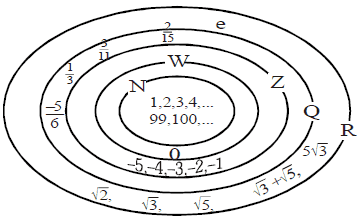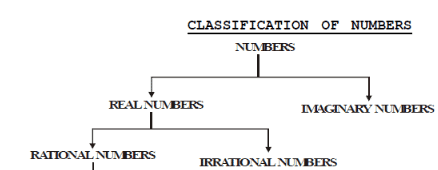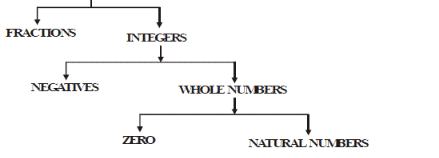Courses

# Real Numbers - Real Numbers, Class 10, Mathematics Class 10 Notes | EduRev

## Class 10 : Real Numbers - Real Numbers, Class 10, Mathematics Class 10 Notes | EduRev

The document Real Numbers - Real Numbers, Class 10, Mathematics Class 10 Notes | EduRev is a part of Class 10 category.
All you need of Class 10 at this link: Class 10

INTRODUCTION
"God gave us the natural number, all else is the work of man". It was exclaimed by L. Kronecker (1823-1891), the reputed German Mathematician. This statement reveals in a nut shell the significant role of the universe of numbers played in the evolution of human thought.

N : The set of natural numbers,

W : The set of whole numbers,

Z : The set of Integers,

Q : The set of rationals,

R : The set of Real NumbersHISTORICAL FACTS

Dedekind was the first modern mathematician to publish in 1872 the mathematically rigorous definition of irrational numbers. He gave explanation of their place in the real Numbers System. He was able to demonstrate the completeness of the real number line. He filled in the 'holes' in the system of Rational numbers with irrational Numbers. This innovation has made Richard Dedekind an immortal figure in the history of Mathematics.

Srinivasa Ramanujan (1887-1920) was one of the most outstanding mathematician that India has produced. He worked on history of Numbers and discovered wonderul properties of numbers. He stated intuitively many complicated result in mathematics. Once a great mathematician Prof. Hardy come to India to see Ramanujan. Prof. Hardy remarked that he has travelled in a taxi with a rather dull number viz. 1729. Ramanujan jumped up and said, Oh! No. 1729 is very interesting number. It is the smallest number which can be expressed as the sum of two cubes in two different ways.
viz 1729 = 13 + 123,
1729 = 93 + 103,
⇒ 1729 = 13 + 123 = 93 + 103

RECALL

In our day to day life, we deal with different types of numbers which can be broadly classified as follows.Natural numbers (N) : N = {1, 2, 3, 4...∞}

 Remark : (i) The set N is infinite i.e. it has unlimited members. (ii) N has the smallest element namely '1'. (iii) N has no largest element. i.e., give me any natural number, we can find the bigger number from the given number. (iv) N does not contain '0' as a member. i.e. '0' is not a member of the set N.

(ii) Whole numbers (W) : W = {0, 1, 2, 3, 4...∞}

 Remark : (i) The set of whole number is infinite (unlimited elements). (ii) This set has the smallest members as '0'. i.e. '0' the smallest whole number. i.e., set W contain '0' as a member. (iii) The set of whole numbers has no largest member. (iv) Every natural number is a whole number. (v) Non-zero smallest whole number is '1'.

(iii) Integers (I or Z) : I or Z = {– ∞... –3, –2, –1, 0, +1, +2, +3 ...+ ∞}

Positive integers : {1, 2, 3...},

Negative integers : {.... –4, –3, –2, –1}

 Remark : (i) This set Z is infinite. (ii) It has neither the greatest nor the least element. (iii) Every natural number is an integer. (iv) Every whole number is an integer. (iv) The set of non-negative integer = {0, 1, 2, 3, 4,....} (v) The set of non-positive integer = {......–4, – 3, – 2, –1, 0}
Offer running on EduRev: Apply code STAYHOME200 to get INR 200 off on our premium plan EduRev Infinity!

,

,

,

,

,

,

,

,

,

,

,

,

,

,

,

,

,

,

,

,

,

,

,

,

,

,

,

;